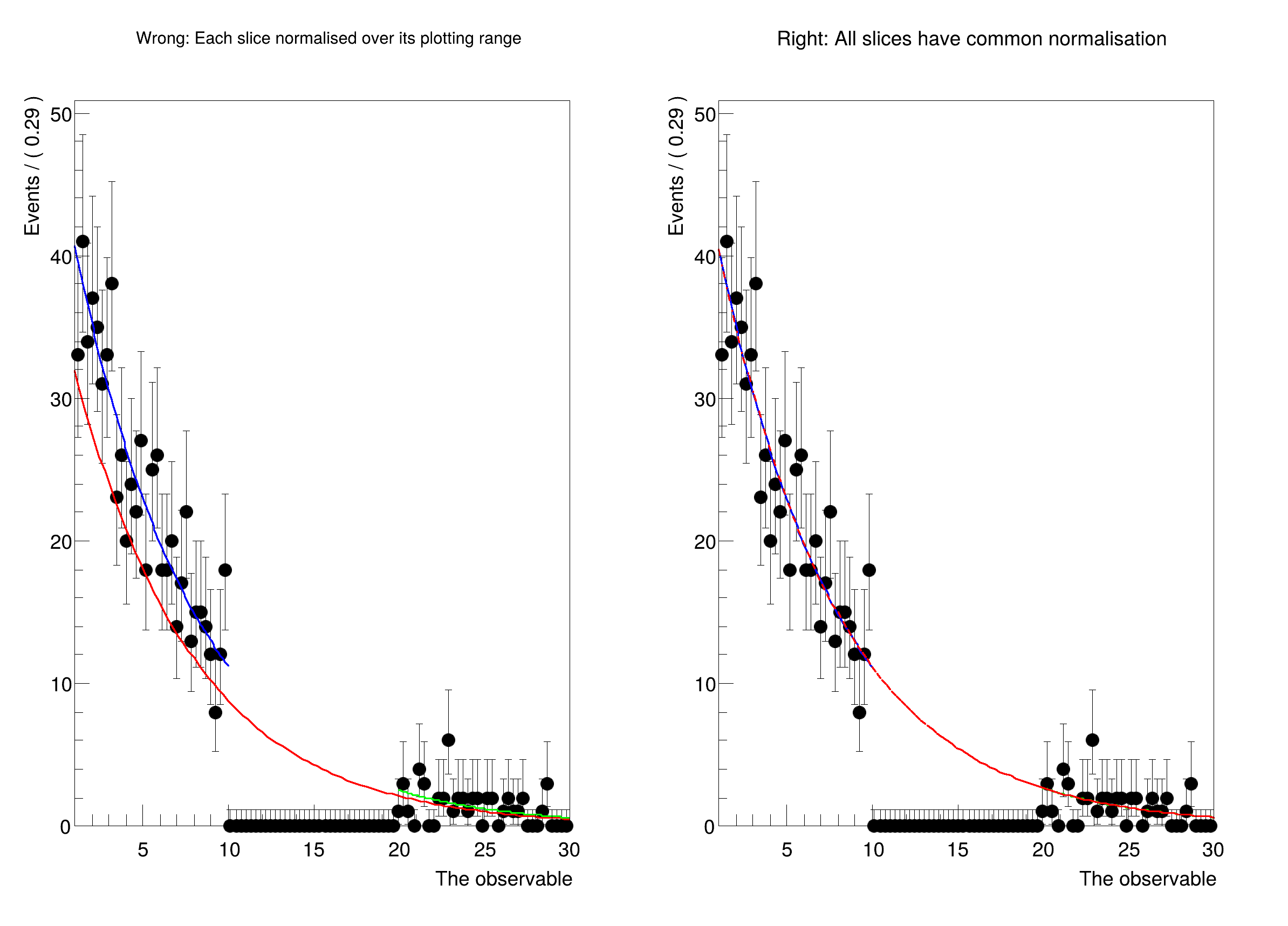ROOT   Reference Guide
Searching...
No Matches
rf212_plottingInRanges_blinding.C File Reference

## Detailed DescriptionPlot a PDF in disjunct ranges, and get normalisation right.

Usually, when comparing a fit to data, one should first plot the data, and then the PDF. In this case, the PDF is automatically normalised to match the number of data events in the plot. However, when plotting only a sub-range, when e.g. a signal region has to be blinded, one has to exclude the blinded region from the computation of the normalisation.

In this tutorial, we show how to explicitly choose the normalisation when plotting using NormRange().

Thanks to Marc Escalier for asking how to do this correctly.#include <RooDataSet.h>
#include <RooExponential.h>
#include <RooPlot.h>
#include <RooRealVar.h>
#include <TCanvas.h>
using namespace RooFit;
{
// Make a fit model
RooRealVar x("x", "The observable", 1, 30);
RooRealVar tau("tau", "The exponent", -0.1337, -10., -0.1);
RooExponential expo("expo", "A falling exponential function", x, tau);
// Define the sidebands (e.g. background regions)
x.setRange("full", 1, 30);
x.setRange("left", 1, 10);
x.setRange("right", 20, 30);
// Generate toy data, and cut out the blinded region.
std::unique_ptr<RooDataSet> data{expo.generate(x, 1000)};
std::unique_ptr<RooAbsData> blindedData{data->reduce(CutRange("left,right"))};
// Kick tau a bit, and run an unbinned fit where the blinded data are missing.
// ----------------------------------------------------------------------------------------------------------
// The fit should be done only in the unblinded regions, otherwise it would
// try to make the model adapt to the empty bins in the blinded region.
tau.setVal(-2.);
expo.fitTo(*blindedData, Range("left,right"), PrintLevel(-1));
// Clear the "fitrange" attribute of the PDF. Otherwise, the fitrange would
// be automatically taken as the NormRange() for plotting. We want to avoid
// this, because the point of this tutorial is to show what can go wrong when
// the NormRange() is not specified.
expo.removeStringAttribute("fitrange");
// Here we will plot the results
TCanvas *canvas=new TCanvas("canvas","canvas",800,600);
canvas->Divide(2,1);
// Wrong:
// ----------------------------------------------------------------------------------------------------------
// Plotting each slice on its own normalises the PDF over its plotting range. For the full curve, that means
// that the blinded region where data is missing is included in the normalisation calculation. The PDF therefore
// comes out too low, and doesn't match up with the slices in the side bands, which are normalised to "their" data.
std::cout << "Now plotting with unique normalisation for each slice." << std::endl;
canvas->cd(1);
RooPlot* plotFrame = x.frame(RooFit::Title("Wrong: Each slice normalised over its plotting range"));
// Plot only the blinded data, and then plot the PDF over the full range as well as both sidebands
blindedData->plotOn(plotFrame);
expo.plotOn(plotFrame, LineColor(kRed), Range("full"));
expo.plotOn(plotFrame, LineColor(kBlue), Range("left"));
expo.plotOn(plotFrame, LineColor(kGreen), Range("right"));
plotFrame->Draw();
// Right:
// ----------------------------------------------------------------------------------------------------------
// Make the same plot, but normalise each piece with respect to the regions "left" AND "right". This requires setting
// a "NormRange", which tells RooFit over which range the PDF has to be integrated to normalise.
// This means that the normalisation of the blue and green curves is slightly different from the left plot,
// because they get a common scale factor.
std::cout << "\n\nNow plotting with correct norm ranges:" << std::endl;
canvas->cd(2);
RooPlot* plotFrameWithNormRange = x.frame(RooFit::Title("Right: All slices have common normalisation"));
// Plot only the blinded data, and then plot the PDF over the full range as well as both sidebands
blindedData->plotOn(plotFrameWithNormRange);
expo.plotOn(plotFrameWithNormRange, LineColor(kBlue), Range("left"), RooFit::NormRange("left,right"));
expo.plotOn(plotFrameWithNormRange, LineColor(kGreen), Range("right"), RooFit::NormRange("left,right"));
expo.plotOn(plotFrameWithNormRange, LineColor(kRed), Range("full"), RooFit::NormRange("left,right"), LineStyle(10));
plotFrameWithNormRange->Draw();
canvas->Draw();
}
@ kRed
Definition Rtypes.h:66
@ kGreen
Definition Rtypes.h:66
@ kBlue
Definition Rtypes.h:66
Option_t Option_t TPoint TPoint const char GetTextMagnitude GetFillStyle GetLineColor GetLineWidth GetMarkerStyle GetTextAlign GetTextColor GetTextSize void data
Exponential PDF.
A RooPlot is a plot frame and a container for graphics objects within that frame.
Definition RooPlot.h:43
static RooPlot * frame(const RooAbsRealLValue &var, double xmin, double xmax, Int_t nBins)
Create a new frame for a given variable in x.
Definition RooPlot.cxx:239
void Draw(Option_t *options=nullptr) override
Draw this plot and all of the elements it contains.
Definition RooPlot.cxx:652
RooRealVar represents a variable that can be changed from the outside.
Definition RooRealVar.h:37
The Canvas class.
Definition TCanvas.h:23
void Draw(Option_t *option="") override
Draw a canvas.
Definition TCanvas.cxx:851
Definition TCanvas.cxx:716
void Divide(Int_t nx=1, Int_t ny=1, Float_t xmargin=0.01, Float_t ymargin=0.01, Int_t color=0) override
RooCmdArg Title(const char *name)
RooCmdArg PrintLevel(Int_t code)
RooCmdArg NormRange(const char *rangeNameList)
RooCmdArg LineColor(Color_t color)
RooCmdArg LineStyle(Style_t style)
Double_t x[n]
Definition legend1.C:17
The namespace RooFit contains mostly switches that change the behaviour of functions of PDFs (or othe...
Ta Range(0, 0, 1, 1)
[#1] INFO:Eval -- RooRealVar::setRange(x) new range named 'full' created with bounds [1,30]
[#1] INFO:Eval -- RooRealVar::setRange(x) new range named 'left' created with bounds [1,10]
[#1] INFO:Eval -- RooRealVar::setRange(x) new range named 'right' created with bounds [20,30]
[#1] INFO:Eval -- RooRealVar::setRange(x) new range named 'fit_nll_expo_expoData_left' created with bounds [1,10]
[#1] INFO:Eval -- RooRealVar::setRange(x) new range named 'fit_nll_expo_expoData_right' created with bounds [20,30]
[#1] INFO:Fitting -- RooAbsOptTestStatistic::ctor(nll_expo_expoData) constructing test statistic for sub-range named left,right
[#1] INFO:Minimization -- RooAbsMinimizerFcn::setOptimizeConst: activating const optimization
[#1] INFO:Minimization -- RooAbsMinimizerFcn::setOptimizeConst: deactivating const optimization
Now plotting with unique normalisation for each slice.
[#1] INFO:Plotting -- RooAbsPdf::plotOn(expo) only plotting range 'full', curve is normalized to data in given range
[#1] INFO:Plotting -- RooAbsPdf::plotOn(expo) only plotting range 'left', curve is normalized to data in given range
[#1] INFO:Plotting -- RooAbsPdf::plotOn(expo) only plotting range 'right', curve is normalized to data in given range
Now plotting with correct norm ranges:
[#1] INFO:Plotting -- RooAbsPdf::plotOn(expo) only plotting range 'left'
[#1] INFO:Plotting -- RooAbsPdf::plotOn(expo) p.d.f. curve is normalized using explicit choice of ranges 'left,right'
[#1] INFO:Plotting -- RooAbsPdf::plotOn(expo) only plotting range 'right'
[#1] INFO:Plotting -- RooAbsPdf::plotOn(expo) p.d.f. curve is normalized using explicit choice of ranges 'left,right'
[#1] INFO:Plotting -- RooAbsPdf::plotOn(expo) only plotting range 'full'
[#1] INFO:Plotting -- RooAbsPdf::plotOn(expo) p.d.f. curve is normalized using explicit choice of ranges 'left,right'
Date
March 2020

Definition in file rf212_plottingInRanges_blinding.C.